Definitions and notation
Patterns In Sequences
Arithmetic sequences and series
Geometric sequences and series
Key Points of this Module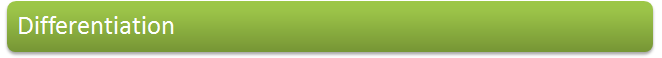Finding the gradient of a curve
Finding the gradient from first principles
Differentiating by using standard results
Tangents and normals
Maximum and minimum points
Increasing and decreasing functions
Stationary Points
Higher derivatives
Applications
Key Points of this Module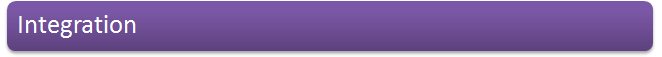Reversing differentiation
Finding the area under the curve
Area as the limit of a sum
Areas below the x axis
The area between two curves
The area between a curve and the y axis
Numerical integration
Key Points of this Module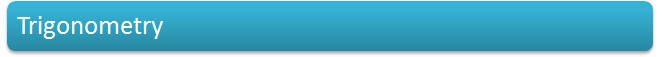Trigonometrical Functions

Key Points of this Module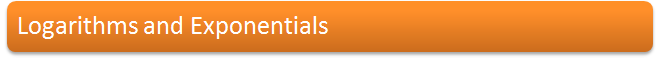Key Points of this Module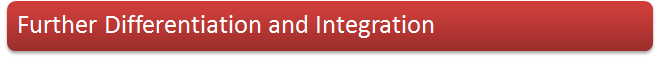Key Points of this ModuleRevision Sheet